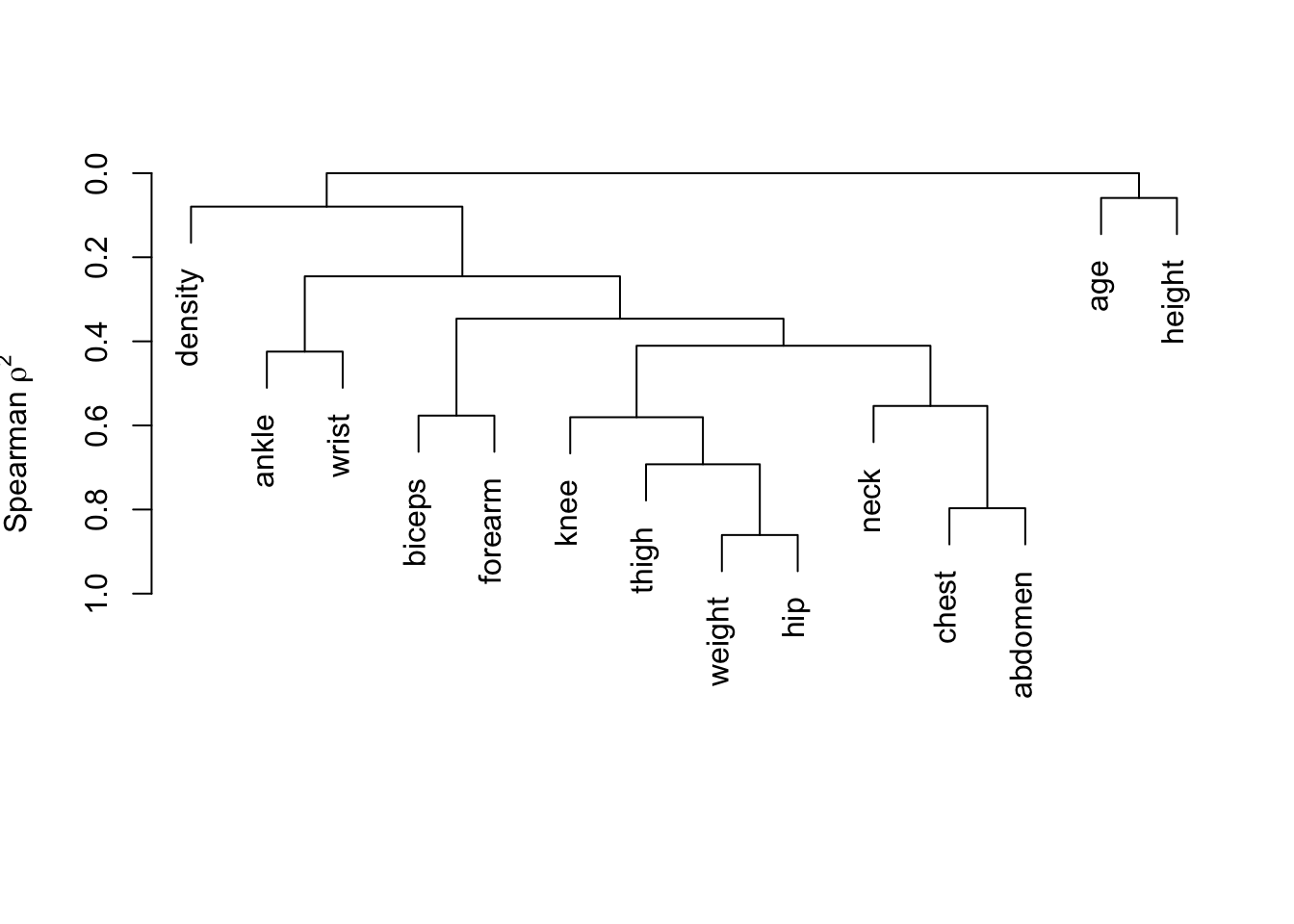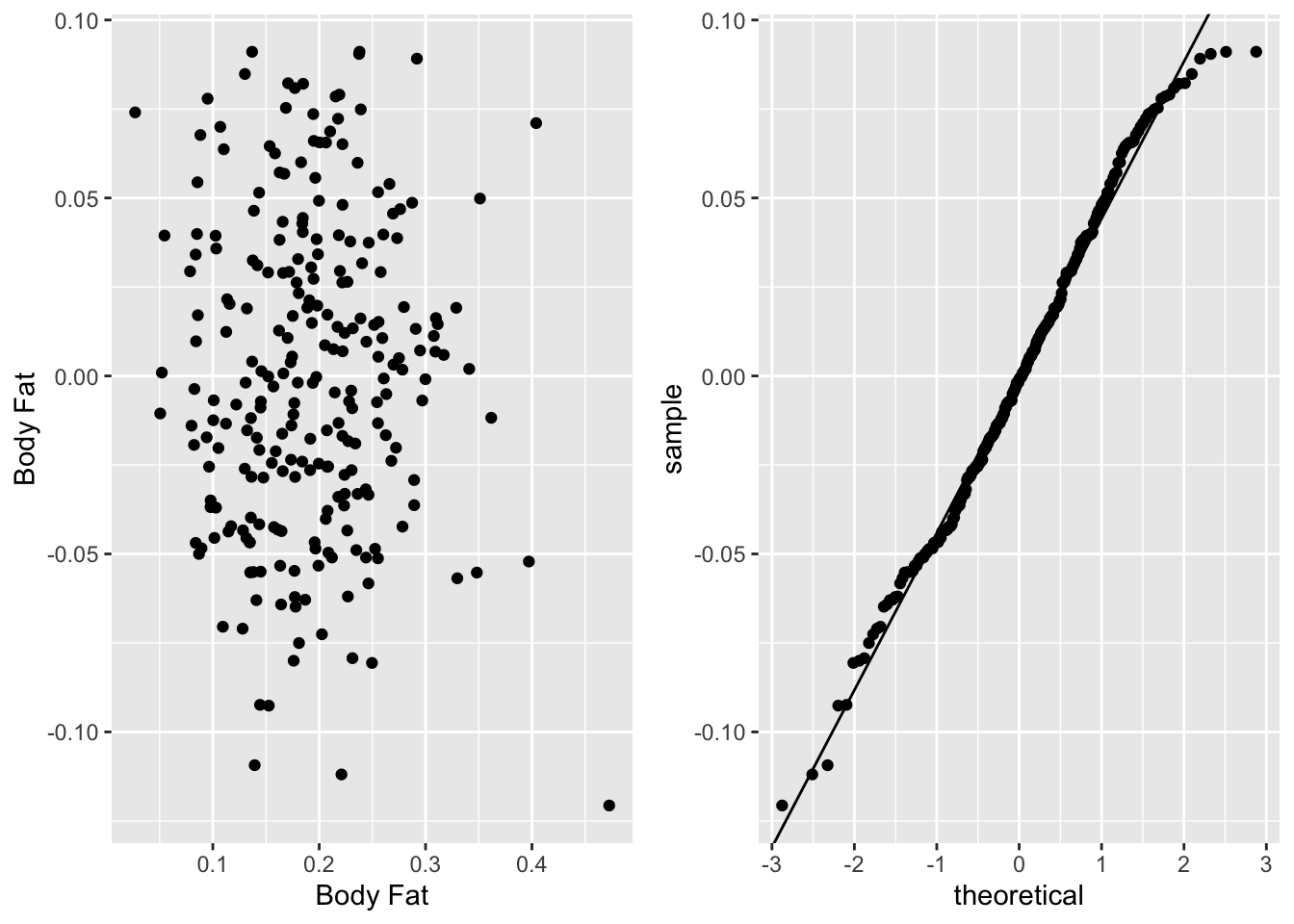# 23  Body Fat: Case Study in Linear Modeling

• Goal: accurately estimate proportion of male adult body mass that is fat using readily available body dimensions and age
• Gold standard body fat was determined by underwater weighing
• Source: kaggle competition
• Original source: Generalized body composition prediction equation for men using simple measurement techniques, K.W. Penrose, A.G. Nelson, A.G. Fisher, FACSM, Human Performance Research Center, Brigham Young University, Provo, Utah 84602 as listed in Medicine and Science in Sports and Exercise, vol. 17, no. 2, April 1985, p. 189
• 252 men; 3 excluded due to erroneous data
• Available at hbiostat.org/data or using getHdata

Statistical Analysis Attack

• Response variable is proportion of body mass that composed of fat
• Display descriptive statistics and variable clustering pattern
• Run a redundancy analysis
• Knowing that abdomen circumference is a dominating predictor and that height must be taken into account when interpreting it, learn about the transformations of the variables from a linear model with predictors abdomen, height, and age
• Compare 4 competing models with AIC
• Assess the contribution of all other size measurements in predicting fat

## 23.1 Descriptive Statistics

Code
getHdata(bodyfat)
d   <- bodyfat
des <- describe(d)
sparkline::sparkline(0)   # load jQuery javascript for sparklines
Code
print(des, which='continuous')
Variable Label Units n Missing Distinct Info Mean Gini |Δ| d Descriptives 15 Continous Variables of 15 Variables, 249 Observations density Density g/cm3 249 0 216 1.000 1.055 0.02119 1.026 1.032 1.041 1.055 1.070 1.080 1.085 fat Body Fat Proportion 249 0 174 1.000 0.1925 0.09364 0.0630 0.0850 0.1250 0.1920 0.2530 0.2992 0.3248 age Age years 249 0 51 0.999 44.95 14.39 25.0 27.0 36.0 43.0 54.0 63.2 67.0 weight Weight kg 249 0 194 1.000 81.39 14.27 62.67 67.17 72.30 80.24 89.44 98.52 102.58 height Height cm 249 0 47 0.999 178.7 7.541 167.9 170.7 174.0 178.4 183.5 187.3 189.2 neck Neck Circumference cm 249 0 89 1.000 38.03 2.66 34.34 35.20 36.40 38.00 39.50 40.92 41.86 chest Chest Circumference cm 249 0 171 1.000 100.9 9.241 89.20 91.36 94.60 99.70 105.30 112.32 116.52 abdomen Abdomen Circumference at Umbilicus cm 249 0 183 1.000 92.67 11.73 77.60 79.68 85.20 91.00 99.20 105.76 110.88 hip Hip Circumference cm 249 0 150 1.000 99.94 7.422 89.36 92.06 95.60 99.30 103.50 108.64 111.86 thigh Thigh Circumference cm 249 0 137 1.000 59.45 5.619 51.52 53.24 56.10 59.00 62.30 65.84 68.46 knee Knee Circumference cm 249 0 89 1.000 38.61 2.638 34.90 35.68 37.10 38.50 39.90 41.70 42.66 ankle Ankle Circumference cm 249 0 60 0.999 23.12 1.694 21.00 21.50 22.00 22.80 24.00 24.82 25.46 biceps Extended Biceps Circumference cm 249 0 103 1.000 32.32 3.363 27.74 28.80 30.30 32.10 34.40 36.24 37.20 forearm Forearm Circumference cm 249 0 76 1.000 28.69 2.235 25.74 26.28 27.30 28.80 30.00 31.10 31.76 wrist Wrist Circumference cm 249 0 44 0.998 18.25 1.039 16.84 17.08 17.60 18.30 18.80 19.40 19.80
Code
plot(varclus(~ . - fat, data=d))Do a redundancy analysis

Code
redun(fat ~ ., data=d)

Redundancy Analysis

fat ~ .

n: 249  p: 15   nk: 3

Number of NAs:   0

Transformation of target variables forced to be linear

R-squared cutoff: 0.9   Type: ordinary

R^2 with which each variable can be predicted from all other variables:

fat density     age  weight  height    neck   chest abdomen     hip   thigh
0.983   0.981   0.605   0.983   0.749   0.788   0.919   0.950   0.938   0.892
knee   ankle  biceps forearm   wrist
0.800   0.502   0.744   0.592   0.769

Rendundant variables:

fat weight abdomen

Predicted from variables:

density age height neck chest hip thigh knee ankle biceps forearm wrist

Variable Deleted   R^2 R^2 after later deletions
1              fat 0.983               0.983 0.982
2           weight 0.983                     0.981
3          abdomen 0.944                          

weight could be dispensed with but we will keep it for historical reasons.

## 23.2 Learn Predictor Transformations and Interactions From Simple Model

• Compare AICs of 5 competing models
• It is safe to use AIC to select from among perhaps 3 models so we are pushing the envelope here
Code
AIC(ols(fat ~ rcs(age, 4) + rcs(height, 4) * rcs(abdomen, 4), data=d))
     d.f.
-825.0106 
Code
AIC(ols(fat ~ rcs(age, 4) + rcs(log(height), 4) + rcs(log(abdomen), 4), data=d))
     d.f.
-833.7197 
Code
AIC(ols(fat ~ rcs(age, 4) + log(height) + log(abdomen), data=d))
     d.f.
-834.4334 
Code
AIC(ols(fat ~ age * (log(height) + log(abdomen)), data=d))
     d.f.
-833.6138 
Code
AIC(ols(fat ~ age + height + abdomen, data=d))
     d.f.
-822.2701 
• Third model has lowest AIC
• Will use its structure when adding other predictors
• Check contant variance and normality assumptions on the winning small model
Code
f <- ols(fat ~ rcs(age, 4) + log(height) + log(abdomen), data=d)
f

Linear Regression Model

ols(formula = fat ~ rcs(age, 4) + log(height) + log(abdomen),
data = d)

Model Likelihood
Ratio Test
Discrimination
Indexes
Obs 249 LR χ2 308.62 R2 0.710
σ 0.0446 d.f. 5 R2adj 0.704
d.f. 243 Pr(>χ2) 0.0000 g 0.077

Residuals

       Min         1Q     Median         3Q        Max
-0.1206613 -0.0331328 -0.0009029  0.0316693  0.0910751 
β S.E. t Pr(>|t|)
Intercept  -0.3810  0.4142 -0.92 0.3586
age   0.0019  0.0010 1.88 0.0610
age'  -0.0053  0.0032 -1.67 0.0960
age''   0.0198  0.0124 1.60 0.1105
height  -0.4357  0.0823 -5.30 <0.0001
abdomen   0.6128  0.0271 22.57 <0.0001
Code
pdx <- function() {
r <- resid(f)
w <- data.frame(r=r, fitted=fitted(f))
p1 <- ggplot(w, aes(x=fitted, y=r)) + geom_point()
p2 <- ggplot(w, aes(sample=r)) + stat_qq() +
geom_abline(intercept=mean(r), slope=sd(r))
gridExtra::grid.arrange(p1, p2, ncol=2)
}
pdx()• Normal and constant variance (across predicted values) on the original fat scale
• Usually would need to transform a [0,1]-restricted variable
• Luckily no predicted values outside [0,1] in the dataset
• Will keep a linear model on untransformed fat

## 23.3 Assess Predictive Discrimination Added by Other Size Variables

Code
g <- ols(fat ~ rcs(age, 4) + log(height) + log(abdomen) + log(weight) + log(neck) +
log(chest) + log(hip) + log(thigh) + log(knee) + log(ankle) + log(biceps) +
log(forearm) + log(wrist), data=d)
AIC(g)
     d.f.
-849.9813 
• By AIC the large model is better
• $$R^{2}_\text{adj}$$ went from 0.704 to 0.733
• How does this translate to improvement in median prediction error?
Code
median(abs(resid(f)))
 0.03283904
Code
median(abs(resid(g)))
 0.02995479
• There is a reduction of 0.003 in the typical prediction error
• Proportion of fat varies from 0.06 - 0.32 (0.05 quantile to 0.95 quantile)
• Additional variables are not worth it
• A typical prediction error of 0.03 makes the model fit for purpose

## 23.4 Model Interpretation

• Image plot
• Nomogram
Code
p <- Predict(f, height, abdomen)
bplot(p, ylabrot=90)Code
plot(nomogram(f), lplab='Fat Fraction')